# 69 Results

View
Selected filters:
• Algebra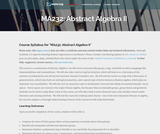Unrestricted Use
CC BY
Rating

This course is a continuation of Abstract Algebra I: the student will revisit structures like groups, rings, and fields as well as mappings like homomorphisms and isomorphisms. The student will also take a look at ring factorization, general lattices, and vector spaces. Later this course presents more advanced topics, such as Galois theory - one of the most important theories in algebra, but one that requires a thorough understanding of much of the content we will study beforehand. Upon successful completion of this course, students will be able to: Compute the sizes of finite groups when certain properties are known about those groups; Identify and manipulate solvable and nilpotent groups; Determine whether a polynomial ring is divisible or not and divide the polynomial (if it is divisible); Determine the basis of a vector space, change bases, and manipulate linear transformations; Define and use the Fundamental Theorem of Invertible Matrices; Use Galois theory to find general solutions of a polynomial over a field. (Mathematics 232)

Subject:
Algebra
Material Type:
Full Course
Provider:
The Saylor Foundation
04/29/2019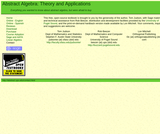Educational Use
Rating

This text is intended for a one- or two-semester undergraduate course in abstract algebra. Traditionally, these courses have covered the theoretical aspects of groups, rings, and fields. However, with the development of computing in the last several decades, applications that involve abstract algebra and discrete mathematics have become increasingly important, and many science, engineering, and computer science students are now electing to minor in mathematics. Though theory still occupies a central role in the subject of abstract algebra and no student should go through such a course without a good notion of what a proof is, the importance of applications such as coding theory and cryptography has grown significantly.

Access also available here: http://abstract.ups.edu/contact.html

Preliminaries
The Integers
Groups
Cyclic Groups
Permutation Groups
Cosets and Lagrange's Theorem
Introduction to Cryptography
Algebraic Coding Theory
Isomorphisms
Normal Subgroups and Factor Groups
Homomorphisms
Matrix Groups and Symmetry
The Structure of Groups
Group Actions
The Sylow Theorems
Rings
Polynomials
Integral Domains
Lattices and Boolean Algebras
Vector Spaces
Fields
Finite Fields
Galois Theory

Subject:
Algebra
Material Type:
Textbook
Provider:
University of Puget Sound
Author:
Thomas Judson
01/01/2016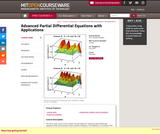Conditional Remix & Share Permitted
CC BY-NC-SA
Rating

" The focus of the course is the concepts and techniques for solving the partial differential equations (PDE) that permeate various scientific disciplines. The emphasis is on nonlinear PDE. Applications include problems from fluid dynamics, electrical and mechanical engineering, materials science, quantum mechanics, etc."

Subject:
Algebra
Material Type:
Full Course
Provider:
M.I.T.
Provider Set:
M.I.T. OpenCourseWare
Author:
Rosales, Rodolfo R.
01/01/2009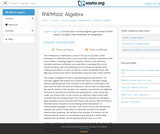Unrestricted Use
CC BY
Rating

This course discusses how to use algebra for a variety of everyday tasks, such as calculate change without specifying how much money is to be spent on a purchase, analyzing relationships by graphing, and describing real-world situations in business, accounting, and science.

Subject:
Algebra
Material Type:
Full Course
Provider:
The Saylor Foundation
04/29/2019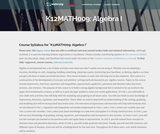Unrestricted Use
CC BY
Rating

This course is oriented toward US high school students. Its structure and materials are aligned to the US Common Core Standards. Foci include: graphing, equations and inequalities.

Subject:
Algebra
Material Type:
Full Course
Provider:
The Saylor Foundation
04/29/2019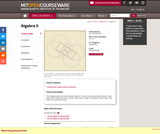Conditional Remix & Share Permitted
CC BY-NC-SA
Rating

This undergraduate level course follows Algebra I. Topics include group representations, rings, ideals, fields, polynomial rings, modules, factorization, integers in quadratic number fields, field extensions, and Galois theory.

Subject:
Algebra
Material Type:
Full Course
Provider:
M.I.T.
Provider Set:
M.I.T. OpenCourseWare
Author:
Artin, Michael
01/01/2011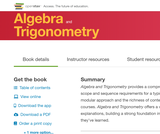Unrestricted Use
CC BY
Rating

Algebra and Trigonometry provides a comprehensive exploration of algebraic principles and meets scope and sequence requirements for a typical introductory algebra and trigonometry course. The modular approach and the richness of content ensures that the book meets the needs of a variety of courses. Algebra and Trigonometry offers a wealth of examples with detailed, conceptual explanations, building a strong foundation in the material before asking students to apply what they’ve learned.

1 Prerequisites
2 Equations and Inequalities
3 Functions
4 Linear Functions
5 Polynomial and Rational Functions
6 Exponential and Logarithmic Functions
7 The Unit Circle: Sine and Cosine Functions
8 Periodic Functions
9 Trigonometric Identities and Equations
10 Further Applications of Trigonometry
11 Systems of Equations and Inequalities
12 Analytic Geometry
13 Sequences, Probability, and Counting Theory

Access also available here: https://openstax.org/details/books/algebra-and-trigonometry

Subject:
Algebra
Trigonometry
Material Type:
Textbook
Provider:
Rice University
Provider Set:
OpenStax College
Author:
David Lippman
Jay Abramson
Jean-Marie Magnier
Melonie Rasmussen
Nicholas Belloit
Rachael Gross
Rick Norwood
Valeree Falduto
01/29/2015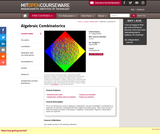Conditional Remix & Share Permitted
CC BY-NC-SA
Rating

" This is an introductory course in algebraic combinatorics. No prior knowledge of combinatorics is expected, but assumes a familiarity with linear algebra and finite groups. Topics were chosen to show the beauty and power of techniques in algebraic combinatorics. Rigorous mathematical proofs are expected."

Subject:
Algebra
Material Type:
Full Course
Provider:
M.I.T.
Provider Set:
M.I.T. OpenCourseWare
Author:
Musiker, Gregg
01/01/2009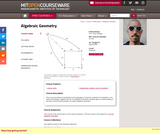Conditional Remix & Share Permitted
CC BY-NC-SA
Rating

" This course provides an introduction to the language of schemes, properties of morphisms, and sheaf cohomology. Together with 18.725 Algebraic Geometry, students gain an understanding of the basic notions and techniques of modern algebraic geometry."

Subject:
Algebra
Geometry
Material Type:
Full Course
Provider:
M.I.T.
Provider Set:
M.I.T. OpenCourseWare
Author:
Kedlaya, Kiran
01/01/2009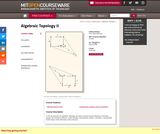Conditional Remix & Share Permitted
CC BY-NC-SA
Rating

In this second term of Algebraic Topology, the topics covered include fibrations, homotopy groups, the Hurewicz theorem, vector bundles, characteristic classes, cobordism, and possible further topics at the discretion of the instructor.

Subject:
Algebra
Material Type:
Full Course
Provider:
M.I.T.
Provider Set:
M.I.T. OpenCourseWare
Author:
Behrens, Mark
01/01/2006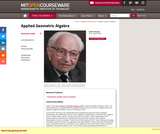Conditional Remix & Share Permitted
CC BY-NC-SA
Rating

Laszlo Tisza was Professor of Physics Emeritus at MIT, where he began teaching in 1941. This online publication is a reproduction the original lecture notes for the course "Applied Geometric Algebra" taught by Professor Tisza in the Spring of 1976. Over the last 100 years, the mathematical tools employed by physicists have expanded considerably, from differential calculus, vector algebra and geometry, to advanced linear algebra, tensors, Hilbert space, spinors, Group theory and many others. These sophisticated tools provide powerful machinery for describing the physical world, however, their physical interpretation is often not intuitive. These course notes represent Prof. Tisza's attempt at bringing conceptual clarity and unity to the application and interpretation of these advanced mathematical tools. In particular, there is an emphasis on the unifying role that Group theory plays in classical, relativistic, and quantum physics. Prof. Tisza revisits many elementary problems with an advanced treatment in order to help develop the geometrical intuition for the algebraic machinery that may carry over to more advanced problems. The lecture notes came to MIT OpenCourseWare by way of Samuel Gasster, '77 (Course 18), who had taken the course and kept a copy of the lecture notes for his own reference. He dedicated dozens of hours of his own time to convert the typewritten notes into LaTeX files and then publication-ready PDFs. You can read about his motivation for wanting to see these notes published in his Preface below. Professor Tisza kindly gave his permission to make these notes available on MIT OpenCourseWare.

Subject:
Algebra
Physics
Material Type:
Full Course
Provider:
M.I.T.
Provider Set:
M.I.T. OpenCourseWare
Author:
Tisza, L
01/01/2009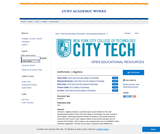Only Sharing Permitted
CC BY-NC-ND
Rating

Arithmetic | Algebra provides a customized open-source textbook for the math developmental students at New York City College of Technology. The book consists of short chapters, addressing essential concepts necessary to successfully proceed to credit-level math courses. Each chapter provides several solved examples and one unsolved “Exit Problem”. Each chapter is also supplemented by its own WeBWork online homework assignment. The book can be used in conjunction with WeBWork for homework (online) or with the Arithmetic | Algebra Homework handbook (traditional). The content in the book, WeBWork and the homework handbook are also aligned to prepare students for the CUNY Elementary Algebra Final Exam (CEAFE).

Subject:
Algebra
Material Type:
Textbook
Provider:
Provider Set:
New York City College of Technology
Author:
Holly Carley
Lin Zhou
Marianna Bonanome
Samar ElHitti
01/01/2017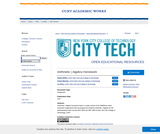Only Sharing Permitted
CC BY-NC-ND
Rating

Arithmetic | Algebra Homework book is a static version of the WeBWork online homework assignments that accompany the textbook Arithmetic | Algebra for the developmental math courses MAT 0630 and MAT 0650 at New York City College of Technology, CUNY.

Subject:
Algebra
Material Type:
Homework/Assignment
Provider:
Provider Set:
New York City College of Technology
Author:
Ariane Masouda
Lin Zhou
Samar ElHitti
01/01/2017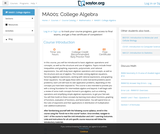Unrestricted Use
CC BY
Rating

This course is also intended to provide the student with a strong foundation for intermediate algebra and beyond. Upon successful completion of this course, you will be able to: simplify and solve linear equations and expressions including problems with absolute values and applications; solve linear inequalities; find equations of lines; and solve application problems; add, subtract, multiply, and divide various types of polynomials; factor polynomials, and simplify square roots; evaluate, simplify, multiply, divide, add, and subtract rational expressions, and solve basic applications of rational expressions. This free course may be completed online at any time. It has been developed through a partnership with the Washington State Board for Community and Technical Colleges; the Saylor Foundation has modified some WSBCTC materials. (Mathematics 001)

Subject:
Algebra
Material Type:
Full Course
Provider:
The Saylor Foundation
04/29/2019Only Sharing Permitted
CC BY-NC-ND
Rating

In this beginning algebra course, you'll learn about
fundamental operations on real numbers
exponents
solving linear equations and inequalities
applications
functions
graphing linear equations
slope
systems of linear equations

Subject:
Algebra
Material Type:
Full Course
Lecture
Module
Provider:
Bossier Parish Community College
Author:
Gail Hendrix
07/15/2020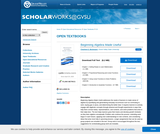Conditional Remix & Share Permitted
CC BY-NC-SA
Rating

Beginning Algebra Made Useful addresses the needs of learners to make sense of algebra by quantifying and generalizing everyday occurrences such as commuting to work, buying gas or pizza, and determining the better deal. It requires learners to actively engage with algebraic concepts through physical and thought experiments in ways that help them connect ideas, representations, and contexts, and solve problems that arise in their daily lives. The text helps learners grow their brains and develop growth mindsets as they learn algebra conceptually. Problem sets continue the process, extending work begun in each lesson, applying new understandings to new contexts, and considering ideas that arise more fully in upcoming lessons. Longer assignments that can be used as group projects are included in the text. Group work is encouraged throughout the text; suggestions for orchestrating group work are included.

The text is open access and free for download by students and instructors in .pdf format. In the electronic format, graphics are in full color and there are live html links to resources, software, and applets.

Chapter 1: Getting Ready to Learn Mathematics
Chapter 2: Proportional Reasoning and Linear Functions
Chapter 3: Using Linear Functions to Solve Problems
Chapter 4: Introduction to Quadratic Functions

Subject:
Mathematics
Algebra
Material Type:
Textbook
Author:
Charlene E. Beckmann
Grand Valley State University
09/08/2021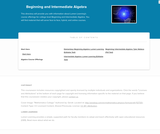Educational Use
Rating

This course covers a range of algebraic topics:  Setting up and solving linear equations, graphing, finding linear relations, solving systems of equations, working with polynomials, factoring, working with rational and radical expressions, solving rational and radical equations, solving quadratic equations, and working with functions.  More importantly, this course is intended to provide you with a solid foundation for the rest of your math courses.  As such, emphasis will be placed on mathematical reasoning, not just memorizing procedures and formulas. There is enough content in this course to cover both beginning and intermediate college-level algebra.

Subject:
Algebra
Material Type:
Full Course
Textbook
Provider:
Lumen Learning
Provider Set:
Candela Courseware
Author:
Tyler Wallace
04/25/2019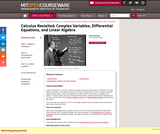Conditional Remix & Share Permitted
CC BY-NC-SA
Rating

Calculus Revisited is a series of videos and related resources that covers the materials normally found in freshman- and sophomore-level introductory mathematics courses. Complex Variables, Differential Equations, and Linear Algebra is the third course in the series, consisting of 20 Videos, 3 Study Guides, and a set of Supplementary Notes. Students should have mastered the first two courses in the series (Single Variable Calculus&nbsp;and&nbsp;Multivariable Calculus) before taking this course. The series was first released in 1972, but equally valuable today for students who are learning these topics for the first time.

Subject:
Algebra
Material Type:
Full Course
Provider:
M.I.T.
Provider Set:
M.I.T. OpenCourseWare
Author:
Herbert Gross
01/01/2011Conditional Remix & Share Permitted
CC BY-NC-SA
Rating

College Algebra is an introductory text for a college algebra survey course. The material is presented at a level intended to prepare students for Calculus while also giving them relevant mathematical skills that can be used in other classes. The authors describe their approach as "Functions First," believing introducing functions first will help students understand new concepts more completely. Each section includes homework exercises, and the answers to most computational questions are included in the text (discussion questions are open-ended).

1 Relations and Functions
3 Polynomial Functions
4 Rational Functions
5 Further Topics in Functions
6 Exponential and Logarithmic Functions
7 Hooked on Conics
8 Systems of Equations and Matrices
9 Sequences and the Binomial Theorem

Subject:
Algebra
Material Type:
Textbook
Provider:
Stitz-Zeager Open Source Mathematics
Author:
Carl Stitz
Jeff Zeager
07/15/2011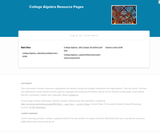Educational Use
Rating

This course covers relations and functions, specifically, linear, polynomial, exponential, logarithmic, and rational functions. Additionally, sections on conics, systems of equations and matrices and sequences are also available.

Subject:
Algebra
Material Type:
Full Course
Textbook
Provider:
Lumen Learning
Provider Set:
Candela Courseware
Author:
Carl Stitz
Jeff Zeager
04/25/2019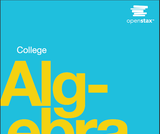Unrestricted Use
CC BY
Rating

College Algebra provides a comprehensive and multi-layered exploration of algebraic principles. The text is suitable for a typical introductory Algebra course, and was developed to be used flexibly. The modular approach and the richness of content ensures that the book meets the needs of a variety of programs.College Algebraguides and supports students with differing levels of preparation and experience with mathematics. Ideas are presented as clearly as possible, and progress to more complex understandings with considerable reinforcement along the way. A wealth of examples – usually several dozen per chapter – offer detailed, conceptual explanations, in order to build in students a strong, cumulative foundation in the material before asking them to apply what they've learned.

OpenStax College has compiled many resources for faculty and students, from faculty-only content to interactive homework and study guides.

Access also available here: https://openstax.org/details/books/college-algebra

1 Prerequisites
2 Equations and Inequalities
3 Functions
4 Linear Functions
5 Polynomial and Rational Functions
6 Exponential and Logarithmic Functions
7 Systems of Equations and Inequalities
8 Analytic Geometry
9 Sequences, Probability, and Counting Theory

Subject:
Algebra
Trigonometry
Material Type:
Textbook
Provider:
Rice University
Provider Set:
OpenStax College
Author:
David Lippman
Jay Abramson
Jean-Marie Magnier
Melonie Rasmussen
Nicholas Belloit
Rachael Gross
Rick Norwood
Valeree Falduto
01/29/2015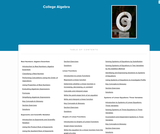Educational Use
Rating

It is often said that mathematics is the language of science. If this is true, then the language of mathematics is numbers. The earliest use of numbers occurred 100 centuries ago in the Middle East to count, or enumerate items. Farmers, cattlemen, and tradesmen used tokens, stones, or markers to signify a single quantitya sheaf of grain, a head of livestock, or a fixed length of cloth, for example. Doing so made commerce possible, leading to improved communications and the spread of civilization.

Subject:
Algebra
Material Type:
Full Course
Textbook
Provider:
Lumen Learning
Provider Set:
Candela Courseware
04/25/2019Only Sharing Permitted
CC BY-NC-ND
Rating

This course is designed to take the concepts you learn in developmental math to expand your knowledge of algebra. This course will focus on two major algebraic concepts to learn - how to SOLVE equations and how to GRAPH equations. Throughout this course you will be challenged to recall ALL of your prior knowledge of operations of real numbers as well as your knowledge related to solving and graphing linear equations (which you should have already mastered from developmental algebra). You will use this prior knowledge to expand on learning the following objectives: solving linear & rational equations. operations of complex numbers, solving quadratic equations, solving radical & polynomial equations, solving equations with rational exponents, solving linear and compound inequalities, solving absolute value equations and inequalities, graphing linear equations & slope, understanding concepts of domain, range and function notation, finding compositions of functions, finding inverses of functions, solving and graphing exponential and logarithmic equations, solving and graphing systems of equations and inequalities, and graphing conics.

*Open Campus courses are non-credit tutorials and cannot, in and of themselves, be used to satisfy degree requirements at Bossier Parish Community College (BPCC). (College Algebra Course by Bossier Parish Community College is licensed under a Creative Commons Attribution-NonCommercial-NoDerivatives 4.0 International License. Based on a work at http://bpcc.edu/opencampus/index.html.)

Subject:
Mathematics
Algebra
Material Type:
Full Course
Provider:
Bossier Parish Community College
Author:
Stacey Black
07/14/2020Conditional Remix & Share Permitted
CC BY-NC-SA
Rating

This book was designed as an introductory trigonometry textbook for college students with the explicit goal of reducing textbook costs.

Subject:
Algebra
Trigonometry
Material Type:
Textbook
Provider:
Stitz-Zeager Open Source Mathematics
Author:
Carl Stitz
Jeff Zeager
07/15/2011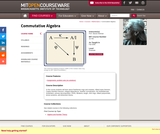Conditional Remix & Share Permitted
CC BY-NC-SA
Rating

In this course students will learn about Noetherian rings and modules, Hilbert basis theorem, Cayley-Hamilton theorem, integral dependence, Noether normalization, the Nullstellensatz, localization, primary decomposition, DVRs, filtrations, length, Artin rings, Hilbert polynomials, tensor products, and dimension theory.

Subject:
Algebra
Material Type:
Full Course
Provider:
M.I.T.
Provider Set:
M.I.T. OpenCourseWare
Author:
Kleiman, Steven
01/01/2008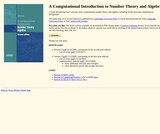Only Sharing Permitted
CC BY-NC-ND
Rating

All of the mathematics required beyond basic calculus is developed “from scratch.” Moreover, the book generally alternates between “theory” and “applications”: one or two chapters on a particular set of purely mathematical concepts are followed by one or two chapters on algorithms and applications; the mathematics provides the theoretical underpinnings for the applications, while the applications both motivate and illustrate the mathematics. Of course, this dichotomy between theory and applications is not perfectly maintained: the chapters that focus mainly on applications include the development of some of the mathematics that is specific to a particular application, and very occasionally, some of the chapters that focus mainly on mathematics include a discussion of related algorithmic ideas as well.

The mathematical material covered includes the basics of number theory (including unique factorization, congruences, the distribution of primes, and quadratic reciprocity) and of abstract algebra (including groups, rings, fields, and vector spaces). It also includes an introduction to discrete probability theory—this material is needed to properly treat the topics of probabilistic algorithms and cryptographic applications. The treatment of all these topics is more or less standard, except that the text only deals with commutative structures (i.e., abelian groups and commutative rings with unity) — this is all that is really needed for the purposes of this text, and the theory of these structures is much simpler and more transparent than that of more general, non-commutative structures.

Access also available here: https://shoup.net/ntb/

1 Basic properties of the integers
2 Congruences
3 Computing with large integers
4 Euclid's algorithm
5 The distribution of primes
6 Abelian groups
7 Rings
8 Finite and discrete probability distributions
9 Probabilistic algorithms
10 Probabilistic primality testing
11 Finding generators and discrete logarithms in Z∗p
12 Quadratic reciprocity and computing modular square roots
13 Modules and vector spaces
14 Matrices
15 Subexponential-time discrete logarithms and factoring
16 More rings
17 Polynomial arithmetic and applications
18 Finite Fields
19 Linearly generated sequences and applications
20 Algorithms for finite fields
21 Deterministic primality testing

Subject:
Mathematics
Algebra
Material Type:
Textbook
Author:
Victor Shoup
01/01/2008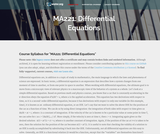Unrestricted Use
CC BY
Rating

This course focuses on linear ordinary differential equations (or ODEs) and will introduce several other subclasses and their respective properties. Despite centuries of study, numerical approximation is the only practical approach to the solution of complicated ODEs that has emerged; this course will introduce you to the fundamentals behind numerical solutions. Upon successful completion of this course, students will be able to: Identify ordinary differential equations and their respective orders; Explain and demonstrate how differential equations are used to model certain situations; Solve first order differential equations as well as initial value problems; Solve linear differential equations with constant coefficients; Use power series to find solutions of linear differential equations, Solve linear systems of differential equations with constant coefficients; Use the Laplace transform to solve initial value problems; Use select methods of numerical approximation to find solutions to differential equations. (Mathematics 221; See also: Mechanical Engineering 003)

Subject:
Algebra
Material Type:
Full Course
Provider:
The Saylor Foundation
04/04/2019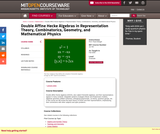Conditional Remix & Share Permitted
CC BY-NC-SA
Rating

" Double affine Hecke algebras (DAHA), also called Cherednik algebras, and their representations appear in many contexts: integrable systems (Calogero-Moser and Ruijsenaars models), algebraic geometry (Hilbert schemes), orthogonal polynomials, Lie theory, quantum groups, etc. In this course we will review the basic theory of DAHA and their representations, emphasizing their connections with other subjects and open problems."

Subject:
Algebra
Geometry
Material Type:
Full Course
Provider:
M.I.T.
Provider Set:
M.I.T. OpenCourseWare
Author:
Etingof, Pavel
01/01/2009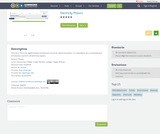Unrestricted Use
CC BY
Rating

This is an algebra-based introductory course for electricity physics. It is intended to be a comprehensive introductory course in all electricity aspects, such as Ohm's Law, AC and DC Circuits, etc.

Subject:
Engineering
Algebra
Physics
Material Type:
Textbook
Author:
03/13/2019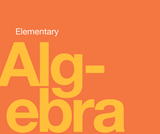Unrestricted Use
CC BY
Rating

Elementary Algebra is designed to meet the scope and sequence requirements of a one-semester elementary algebra course. The book’s organization makes it easy to adapt to a variety of course syllabi. The text expands on the fundamental concepts of algebra while addressing the needs of students with diverse backgrounds and learning styles. Each topic builds upon previously developed material to demonstrate the cohesiveness and structure of mathematics.

1. Foundations
2. Solving Linear Equations and Inequalities
3. Math Models
4. Graphs
5. Systems of Linear Equations
6. Polynomials
7. Factoring
8. Rational Expressions and Equations

Access also available here: https://openstax.org/details/books/elementary-algebra

Subject:
Mathematics
Algebra
Material Type:
Textbook
Author:
Lynn Marecek
MaryAnne Anthony-Smith
03/28/2019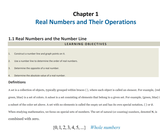Conditional Remix & Share Permitted
CC BY-NC-SA
Rating

This text respects the traditional approaches to algebra pedagogy while enhancing it with the technology available today. In addition, textual notation is introduced as a means to communicate solutions electronically throughout the text. While it is important to obtain the skills to solve problems correctly, it is just as important to communicate those solutions with others effectively in the modern era of instant communications.While algebra is one of the most diversely applied subjects, students often find it to be one of the more difficult hurdles in their education. With this in mind, John wrote Elementary Algebra from the ground up in an open and modular format, allowing the instructor to modify it and leverage their individual expertise as a means to maximize the student experience and success. Elementary Algebra takes the best of the traditional, practice-driven algebra texts and combines it with modern amenities to influence learning, like online/inline video solutions, as well as, other media driven features that only a free online text can deliver.

Chapter 1: Real Numbers and Their Operations
Chapter 2: Linear Equations and Inequalities
Chapter 3: Graphing Lines
Chapter 4: Solving Linear Systems
Chapter 5: Polynomials and Their Operations
Chapter 6: Factoring and Solving by Factoring
Chapter 7: Rational Expressions and Equations
Chapter 8: Radical Expressions and Equations
Chapter 9: Solving Quadratic Equations and Graphing Parabolas
Chapter 10: Appendix: Geometric Figures

Subject:
Algebra
Material Type:
Textbook
Provider:
The Saylor Foundation
Provider Set:
Saylor Textbooks
Author:
John Redden
01/01/2011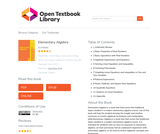Unrestricted Use
CC BY
Rating

Elementary Algebra is a work text that covers the traditional topics studied in a modern elementary algebra course. Use of this book will help the student develop the insight and intuition necessary to master algebraic techniques and manipulative skills.Elementary Algebra is a work text that covers the traditional topics studied in a modern elementary algebra course. It is intended for students who (1) have no exposure to elementary algebra, (2) have previously had an unpleasant experience with elementary algebra, or (3) need to review algebraic concepts and techniques.

1 Arithmetic Review
2 Basic Properties of Real Numbers
3 Basic Operations with Real Numbers
4 Algebraic Expressions and Equations
5 Solving Linear Equations and Inequalities
6 Factoring Polynomials
7 Graphing Linear Equations and Inequalities in One and Two Variables
8 Rational Expressions
9 Roots, Radicals, and Square Root Equations
11 Systems of Linear Equations
12 Appendix

Subject:
Algebra
Material Type:
Textbook
Author:
Denny Burzynski
06/26/2020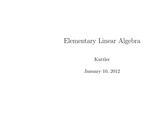Unrestricted Use
CC BY
Rating

Elementary Linear Algebra was written and submitted to the Open Textbook Challenge by Dr. Kenneth Kuttler of Brigham Young University. Dr. Kuttler wrote this textbook for use by his students at BYU. According to the introduction of Elementary Linear Algebra, ĺÎĺ_ĺĚĄ_this is intended to be a first course in linear algebra for students who are sophomores or juniors who have had a course in one variable calculus and a reasonable background in college algebra.ĺÎĺ_ĺĚĺÎĺ A solutions manual for the textbook is included.

Subject:
Algebra
Material Type:
Textbook
Provider:
The Saylor Foundation
Provider Set:
Saylor Textbooks
Author:
Kenneth Kuttler
04/24/2019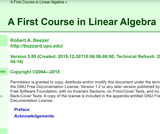Conditional Remix & Share Permitted
CC BY-SA
Rating

A First Course in Linear Algebra is an introductory textbook aimed at college-level sophomores and juniors. Typically students will have taken calculus, but it is not a prerequisite. The book begins with systems of linear equations, then covers matrix algebra, before taking up finite-dimensional vector spaces in full generality. The final chapter covers matrix representations of linear transformations, through diagonalization, change of basis and Jordan canonical form. Determinants and eigenvalues are covered along the way.

Systems of Linear Equations
Vectors
Matrices
Vector Spaces
Determinants
Eigenvalues
Linear Transformations
Representations
Preliminaries
Reference

Access also available here: http://linear.ups.edu/

Subject:
Mathematics
Algebra
Material Type:
Textbook
Provider:
University of Puget Sound
Author:
Robert Beezer
04/24/2019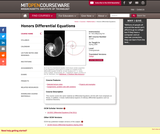Conditional Remix & Share Permitted
CC BY-NC-SA
Rating

"This course covers the same material as Differential Equations (18.03) with more emphasis on theory. In addition, it treats mathematical aspects of ordinary differential equations such as existence theorems."

Subject:
Algebra
Material Type:
Full Course
Provider:
M.I.T.
Provider Set:
M.I.T. OpenCourseWare
Author:
Hur, Vera Mikyoung
01/01/2009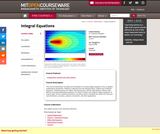Conditional Remix & Share Permitted
CC BY-NC-SA
Rating

Selection of material from the following topics: calculus of variations (the first variation and the second variation); integral equations (Volterra equations; Fredholm equations, the Hilbert-Schmidt theorem); the Hilbert Problem and singular integral equations of Cauchy type; Wiener-Hopf Method and partial differential equations; Wiener-Hopf Method and integral equations; group theory.

Subject:
Algebra
Material Type:
Full Course
Provider:
M.I.T.
Provider Set:
M.I.T. OpenCourseWare
Author:
Margetis, Dionisios
01/01/2006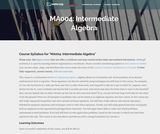Unrestricted Use
CC BY
Rating

This course is a continuation of MA001: Beginning Algebra, and will focus on compound inequalities, systems of linear equations, radicals, rational exponents, quadratic equations and techniques used to solve these equations, and finally, general functions and graphs with an emphasis on the exponential and logarithmic functions.

Subject:
Algebra
Material Type:
Full Course
Provider:
The Saylor Foundation
04/29/2019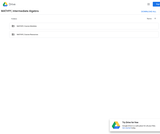Unrestricted Use
CC BY
Rating

Students will learn to solve compound inequalities, absolute value inequalities, and systems of equations, simplify radical expressions, solve quadratic equations and applications and simplify compound fractions, solve rational equations and applications, use function notation to solve problems and use exponential and logarithmic functions.Login: guest_oclPassword: ocl

Subject:
Algebra
Material Type:
Full Course
Homework/Assignment
Lecture Notes
Syllabus
Provider:
Washington State Board for Community & Technical Colleges
Provider Set:
Open Course Library
10/31/2011Conditional Remix & Share Permitted
CC BY-NC-SA
Rating

A community college level textbook that provides non-STEM students with a high-quality and academically rigorous book.

Subject:
Algebra
Material Type:
Textbook
Provider:
Brookdale Community College
Author:
Barbara Tozzi
Linda Wang
07/14/2020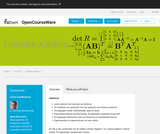Conditional Remix & Share Permitted
CC BY-NC-SA
Rating

Vakinhoud:
- Leren rekenen met vectoren en matrices.
- De methode van rijreductie voor het oplossen van lineaire systemen.
- De begrippen lineair onafhankelijk, span en basis
- Elementaire lineaire transformaties, de begrippen surjectief en injectief.
- De begrippen deelruimte, basis en dimensie en voorbeelden hiervan.
- Eigenwaardes en eigenvectoren van een matrix.
- Dit vak is een combinatie van de vakken Lineaire Algebra 1 en Lineaire Algebra 2 die bij andere TU-opleidingen aangeboden worden.

Leerdoelen:
- Het kennen van basisbegrippen, het gebruik van basismethodes.
- Het maken van logische afleidingen met behulp van deze begrippen en methodes

Subject:
Algebra
Material Type:
Assessment
Homework/Assignment
Lecture
Lecture Notes
Provider:
Delft University of Technology
Provider Set:
Delft University OpenCourseWare
Author:
Dr. B.J. Meulenbroek
04/25/2019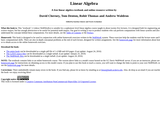Conditional Remix & Share Permitted
CC BY-NC-SA
Rating

We believe the entire book can be taught in twenty five 50-minute lectures to a sophomore audience that has been exposed to a one year calculus course. Vector calculus is useful, but not necessary preparation for this book, which attempts to be self-contained. Key concepts are presented multiple times, throughout the book, often first in a more intuitive setting, and then again in a definition, theorem, proof style later on. We do not aim for students to become agile mathematical proof writers, but we do expect them to be able to show and explain why key results hold. We also often use the review exercises to let students discover key results for themselves; before they are presented again in detail later in the book.

Subject:
Algebra
Material Type:
Textbook
Author:
Andrew Waldon
David Cherney
Tom Denton
01/01/2016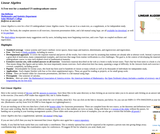Conditional Remix & Share Permitted
CC BY-SA
Rating

Chapter One: Solving Linear Systems
Chapter Two: Vector Spaces
Chapter Three: Maps Between Spaces
Chapter Four: Determinants
Chapter Five: Similarity

This text covers the standard material for a US undergraduate first course: linear systems and Gauss's Method, vector spaces, linear maps and matrices, determinants, and eigenvectors and eigenvalues, as well as additional topics such as introductions to various applications. It has extensive exercise sets with worked answers to all exercises, including proofs, beamer slides for classroom use, and a lab manual for computer work. The approach is developmental. Although everything is proved, it introduces the material with a great deal of motivation, many computational examples, and exercises that range from routine verifications to a few challenges.

Access also available here: http://joshua.smcvt.edu/linearalgebra/

Subject:
Algebra
Material Type:
Textbook
Provider:
St. Michael's College
Author:
Jim Hefferon
01/01/2011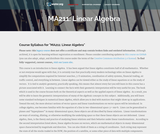Unrestricted Use
CC BY
Rating

This course introduces the student to the study of linear algebra. Practically every modern technology relies on linear algebra to simplify the computations required for internet searches, 3-D animation, coordination of safety systems, financial trading, air traffic control, and everything in between. Upon completion of this course, the student will be able to: Define and identify linear equations; Write a system of equations in matrix-vector form; Explain the geometric interpretation of a system of linear equations; Solve linear equations using a variety of methods; Define general, particular, and homogeneous solutions; Identify how many solutions a linear system has; Correctly manipulate vectors algebraically and perform matrix-vector and matrix-matrix multiplication; Define linear combination and span; Define and distinguish between singular and nonsingular matrices and calculate a matrix inverse; Define and compute LU decompositions; Relate invertibility of matrices to solvability of linear systems; Define and characterize Euclidean space; Define and compute dot and cross-products; Define and identify vector spaces and subspaces; Define spanning set and determine the span of a set of vectors; Define and verify linear independence; Define basis and dimension; Show that a set of vectors is a basis; Define and compute column space, row space, nullspace, and rank; Define and identify isomorphisms and homomorphisms; Use row and column space to solve linear systems; State the rank-nullity theorem; Define inner product, inner product space, and orthogonality; Interpret inner products geometrically; Define determinants using the permutation expansion; State the properties of determinants, such as that the determinant of the product is the product of the determinants; Compute the determinant using cofactor expansions, row reduction, and Cramer's Rule; Define and compute the characteristic polynomial of a matrix; Define and compute eigenvalues and eigenvectors; Explain the geometric significance of eigenvalues and eigenvectors; Define similarity and diagonalizability; Identify similar matrices; Identify some necessary conditions for diagonalizability. (Mathematics 211; See also: Computer Science 105)

Subject:
Algebra
Material Type:
Full Course
Provider:
The Saylor Foundation
04/29/2019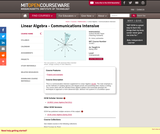Conditional Remix & Share Permitted
CC BY-NC-SA
Rating

This is a communication intensive supplement to Linear Algebra (18.06). The main emphasis is on the methods of creating rigorous and elegant proofs and presenting them clearly in writing.

Subject:
Algebra
Material Type:
Full Course
Provider:
M.I.T.
Provider Set:
M.I.T. OpenCourseWare
Author:
Lachowska, Anna (liakhovskaia)
01/01/2004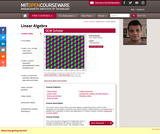Conditional Remix & Share Permitted
CC BY-NC-SA
Rating

This course covers matrix theory and linear algebra, emphasizing topics useful in other disciplines such as physics, economics and social sciences, natural sciences, and engineering.

Subject:
Algebra
Material Type:
Full Course
Provider:
M.I.T.
Provider Set:
M.I.T. OpenCourseWare
Author:
Strang, Gilbert
01/01/2011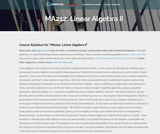Unrestricted Use
CC BY
Rating

Linear Algebra is both rich in theory and full of interesting applications; in this course the student will try to balance both. This course includes a review of topics learned in Linear Algebra I. Upon successful completion of this course, the student will be able to: Solve systems of linear equations; Define the abstract notions of vector space and inner product space; State examples of vector spaces; Diagonalize a matrix; Formulate what a system of linear equations is in terms of matrices; Give an example of a space that has the Archimedian property; Use the Euclidean algorithm to find the greatest common divisor; Understand polar form and geometric interpretation of the complex numbers; Explain what the fundamental theorem of algebra states; Determine when two matrices are row equivalent; State the Fredholm alternative; Identify matrices that are in row reduced echelon form; Find a LU factorization for a given matrix; Find a PLU factorization for a given matrix; Find a QR factorization for a given matrix; Use the simplex algorithm; Compute eigenvalues and eigenvectors; State Shur's Theorem; Define normal matrices; Explain the composition and the inversion of permutations; Define and compute the determinant; Explain when eigenvalues exist for a given operator; Normal form of a nilpotent operator; Understand the idea of Jordan blocks, Jordan matrices, and the Jordan form of a matrix; Define quadratic forms; State the second derivative test; Define eigenvectors and eigenvalues; Define a vector space and state its properties; State the notions of linear span, linear independence, and the basis of a vector space; Understand the ideas of linear independence, spanning set, basis, and dimension; Define a linear transformation; State the properties of linear transformations; Define the characteristic polynomial of a matrix; Define a Markov matrix; State what it means to have the property of being a stochastic matrix; Define a normed vector space; Apply the Cauchy Schwarz inequality; State the Riesz representation theorem; State what it means for a nxn matrix to be diagonalizable; Define Hermitian operators; Define a Hilbert space; Prove the Cayley Hamilton theorem; Define the adjoint of an operator; Define normal operators; State the spectral theorem; Understand how to find the singular-value decomposition of an operator; Define the notion of length for abstract vectors in abstract vector spaces; Define orthogonal vectors; Define orthogonal and orthonormal subsets of R^n; Use the Gram-Schmidt process; Find the eigenvalues and the eigenvectors of a given matrix numerically; Provide an explicit description of the Power Method. (Mathematics 212)

Subject:
Algebra
Material Type:
Full Course
Provider:
The Saylor Foundation
04/29/2019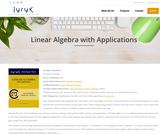Conditional Remix & Share Permitted
CC BY-NC-SA
Rating

After being traditionally published for many years, this formidable text by W. Keith Nicholson is now being released as an open educational resource and part of Lyryx with Open Texts! Supporting today’s students and instructors requires much more than a textbook, which is why Dr. Nicholson opted to work with Lyryx Learning.

Overall, the aim of the text is to achieve a balance among computational skills, theory, and applications of linear algebra. It is a relatively advanced introduction to the ideas and techniques of linear algebra targeted for science and engineering students who need to understand not only how to use these methods but also gain insight into why they work.

The contents have enough flexibility to present a traditional introduction to the subject, or to allow for a more applied course. Chapters 1–4 contain a one-semester course for beginners whereas Chapters 5–9 contain a second semester course. The text is primarily about real linear algebra with complex numbers being mentioned when appropriate (reviewed in Appendix A).

Reviews available here: https://open.umn.edu/opentextbooks/textbooks/linear-algebra-with-applications

Subject:
Algebra
Material Type:
Textbook
Provider:
Lyryx Learning
Author:
W. Keith Nicholson
04/24/2019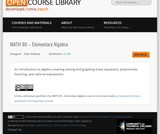Unrestricted Use
CC BY
Rating

An introduction to algebra covering solving and graphing linear equations, polynomials, factoring, and rational expressions

Subject:
Algebra
Material Type:
Full Course
Author:
Tyler Wallace
11/30/2020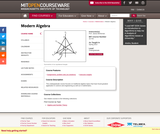Conditional Remix & Share Permitted
CC BY-NC-SA
Rating

This undergraduate course focuses on traditional algebra topics that have found greatest application in science and engineering as well as in mathematics.

Subject:
Algebra
Material Type:
Full Course
Provider:
M.I.T.
Provider Set:
M.I.T. OpenCourseWare
Author:
James McKernan
01/01/2013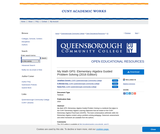Conditional Remix & Share Permitted
CC BY-NC-SA
Rating

My Math GPS: Elementary Algebra Guided Problem Solving is a textbook that aligns to the CUNY Elementary Algebra Learning Objectives that are tested on the CUNY Elementary Algebra Final Exam (CEAFE). This book contextualizes arithmetic skills into Elementary Algebra content using a problem-solving pedagogy. Classroom assessments and online homework are available from the authors.

Linear Equations And Inequalities

GPS 1: Understanding Linear Equations
GPS 2: Solving Linear Equations With Whole Numbers
GPS 4: Subtracting Signed Numbers
GPS 5: Multiplying And Dividing Signed Numbers
GPS 6: Solving Linear Equations With Signed Numbers
GPS 7: Translating Words Into Expressions And Equations
GPS 8: Solving Linear Inequalities, Part 1
GPS 9: Solving Linear Inequalities, Part 2
GPS 10: Solving Linear Equations With Fractions
GPS 11: More Linear Equations With Fractions
GPS 12: Solving Literal Linear Equations
The Coordinate Plane And Lines

GPS 13: Introduction To The Coordinate Plane
GPS 14: Intercepts Of A Line
GPS 15: Slope And Equations Of A Line
GPS 16: The Slope-Intercept Equation Of A Line
GPS 17: Slope-Intercept Equation And Graphing
GPS 18: Graphing Lines In Slope-Intercept Form
GPS 19: Finding An Equation Of A Line From Its Graph
GPS 20: Horizontal And Vertical Lines
Systems Of Linear Equations

GPS 21: Introduction To Systems Of Linear Equations And Solving Graphically
GPS 22: More On Solving Systems Graphically
GPS 23: Solving Systems Of Linear Equations Algebraically
GPS 24: More On Solving Systems Algebraically
Exponents

GPS 25: Rules Of Exponents, Part 1
GPS 26: Rules Of Exponents, Part 2
Polynomials And Operations

GPS 27: Introduction To Polynomials And Operations
GPS 28: Multiplying Polynomials
GPS 29: Removing The Greatest Common Factor
GPS 30: Factoring By Grouping
GPS 31: Factoring Trinomials By Grouping – Part 1
GPS 32: Factoring Trinomials By Grouping – Part 2
GPS 33: Factoring Trinomials By Grouping – Part 3
GPS 34: Factoring Trinomials By Grouping – Part 4
GPS 35: Factoring A Difference Of Squares
GPS 36: Multistep Factoring
GPS 37: Solving Quadratic Equations By Factoring
Algebraic Expressions

GPS 38: Evaluating Algebraic Expressions
Square Roots And Operations

GPS 39: Introduction To Square Roots
GPS 40: Operations With Square Roots
GPS 41: Pythagorean Theorem

Subject:
Algebra
Material Type:
Textbook
Provider:
Provider Set:
Queensborough Community College
Author:
G. Michael Guy
Jonathan Cornick
Karan Puri
01/01/2016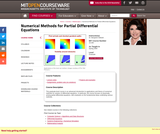Conditional Remix & Share Permitted
CC BY-NC-SA
Rating

" This graduate-level course is an advanced introduction to applications and theory of numerical methods for solution of differential equations. In particular, the course focuses on physically-arising partial differential equations, with emphasis on the fundamental ideas underlying various methods."

Subject:
Algebra
Material Type:
Full Course
Provider:
M.I.T.
Provider Set:
M.I.T. OpenCourseWare
Author:
Seibold, Benjamin
01/01/2009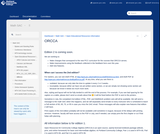Unrestricted Use
CC BY
Rating

This textbook, winner of the 2018 Digital Learning Innovation Award, was developed by the mathematics faculty at Portland Community College under the direction of Alex Jordan and Ann Cary with careful attention to accessibility issues. It has been class tested there and is being used elsewhere. The book is offered in three formats: online HTML (or eBook), PDF, and print. The versions are essentially identical, but the eBook version has features unavailable in the other versions such as the video lessons and checkpoint problems with boxes for the student to enter answers and submit them for checking (not grading). In the PDF and print versions the checkpoint problems are followed by worked out solutions.

The various versions are all produced from the same source files written in PreTeXt.

Basic Math Review
Variables, Expressions, and Equations
Linear Equations and Inequalities
Graphing Lines
Systems of Linear Equations
Exponents and Polynomials
Factoring
Functions and Their Representations
Absolute Value Functions
Rational Functions and Equations

Other formats and reviews available here: https://open.umn.edu/opentextbooks/textbooks/open-resources-for-community-college-algebra

Subject:
Mathematics
Algebra
Material Type:
Textbook
Author:
Alex Jordan
Ann Cary
07/11/2019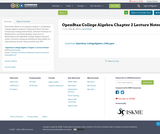Conditional Remix & Share Permitted
CC BY-NC-SA
Rating

PowerPoint Slides to accompany Chapter 2 of OpenStax College Algebra textbook. Prepared by River Parishes Community College (Jared Eusea, Assistant Professor of Mathematics, and Ginny Bradley, Instructor of Mathematics) for OpenStax College Algebra textbook under a Creative Commons Attribution-ShareAlike 4.0 International License. Date provided: July 2019.

Subject:
Algebra
Material Type:
Lecture
Lecture Notes
Author:
Jared Eusea
07/30/2019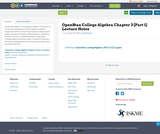Conditional Remix & Share Permitted
CC BY-NC-SA
Rating

PowerPoint Slides to accompany Chapter 3 (Sections 3.1, 3.2 and 3.3) of OpenStax College Algebra textbook. Prepared by River Parishes Community College (Jared Eusea, Assistant Professor of Mathematics, and Ginny Bradley, Instructor of Mathematics) for OpenStax College Algebra textbook under a Creative Commons Attribution-ShareAlike 4.0 International License. Date provided: July 2019.

Subject:
Algebra
Material Type:
Lecture
Lecture Notes
Author:
Jared Eusea
07/30/2019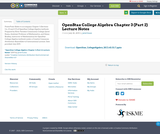Conditional Remix & Share Permitted
CC BY-NC-SA
Rating

PowerPoint Slides to accompany Chapter 3 (Sections 3.4, 3.5 and 3.7) of OpenStax College Algebra textbook. Prepared by River Parishes Community College (Jared Eusea, Assistant Professor of Mathematics, and Ginny Bradley, Instructor of Mathematics) for OpenStax College Algebra textbook under a Creative Commons Attribution-ShareAlike 4.0 International License. Date provided: July 2019.

Subject:
Algebra
Material Type:
Lecture
Lecture Notes
Author:
Jared Eusea
07/30/2019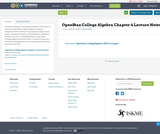Conditional Remix & Share Permitted
CC BY-NC-SA
Rating

PowerPoint Slides to accompany Chapter 4 (Sections 4.1 and 4.2) of OpenStax College Algebra textbook. Prepared by River Parishes Community College (Jared Eusea, Assistant Professor of Mathematics, and Ginny Bradley, Instructor of Mathematics) for OpenStax College Algebra textbook under a Creative Commons Attribution-ShareAlike 4.0 International License. Date provided: July 2019.

Subject:
Algebra
Material Type:
Lecture
Lecture Notes
Author:
Jared Eusea
07/30/2019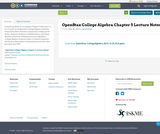Conditional Remix & Share Permitted
CC BY-NC-SA
Rating

PowerPoint Slides to accompany Chapter 5 (Sections 5.1, 5.2, 5.3 and 5.6) of OpenStax College Algebra textbook. Prepared by River Parishes Community College (Jared Eusea, Assistant Professor of Mathematics, and Ginny Bradley, Instructor of Mathematics) for OpenStax College Algebra textbook under a Creative Commons Attribution-ShareAlike 4.0 International License. Date provided: July 2019.

Subject:
Algebra
Material Type:
Lecture
Lecture Notes
Author:
Jared Eusea
07/30/2019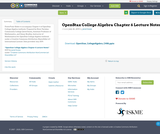Conditional Remix & Share Permitted
CC BY-NC-SA
Rating

PowerPoint Slides to accompany Chapter 6 of OpenStax College Algebra textbook. Prepared by River Parishes Community College (Jared Eusea, Assistant Professor of Mathematics, and Ginny Bradley, Instructor of Mathematics) for OpenStax College Algebra textbook under a Creative Commons Attribution-ShareAlike 4.0 International License. Date provided: July 2019.

Subject:
Algebra
Material Type:
Lecture
Lecture Notes
Author:
Jared Eusea
07/30/2019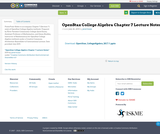Conditional Remix & Share Permitted
CC BY-NC-SA
Rating

PowerPoint Slides to accompany Chapter 7 (Section 7.1 only) of OpenStax College Algebra textbook. Prepared by River Parishes Community College (Jared Eusea, Assistant Professor of Mathematics, and Ginny Bradley, Instructor of Mathematics) for OpenStax College Algebra textbook under a Creative Commons Attribution-ShareAlike 4.0 International License. Date provided: July 2019.

Subject:
Algebra
Material Type:
Lecture
Lecture Notes
Author:
Jared Eusea
07/30/2019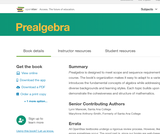Unrestricted Use
CC BY
Rating

Prealgebra is designed to meet scope and sequence requirements for a one-semester prealgebra course. The book’s organization makes it easy to adapt to a variety of course syllabi. The text introduces the fundamental concepts of algebra while addressing the needs of students with diverse backgrounds and learning styles. Each topic builds upon previously developed material to demonstrate the cohesiveness and structure of mathematics.

1 Whole Numbers
2 The Language of Algebra
3 Integers
4 Fractions
5 Decimals
6 Percents
7 The Properties of Real Numbers
8 Solving Linear Equations
9 Math Models and Geometry
10 Polynomials
11 Graphs

Access also available here: https://openstax.org/details/books/prealgebra

Subject:
Algebra
Material Type:
Textbook
Provider:
Rice University
Provider Set:
OpenStax College
Author:
MaryAnne Anthony-Smith